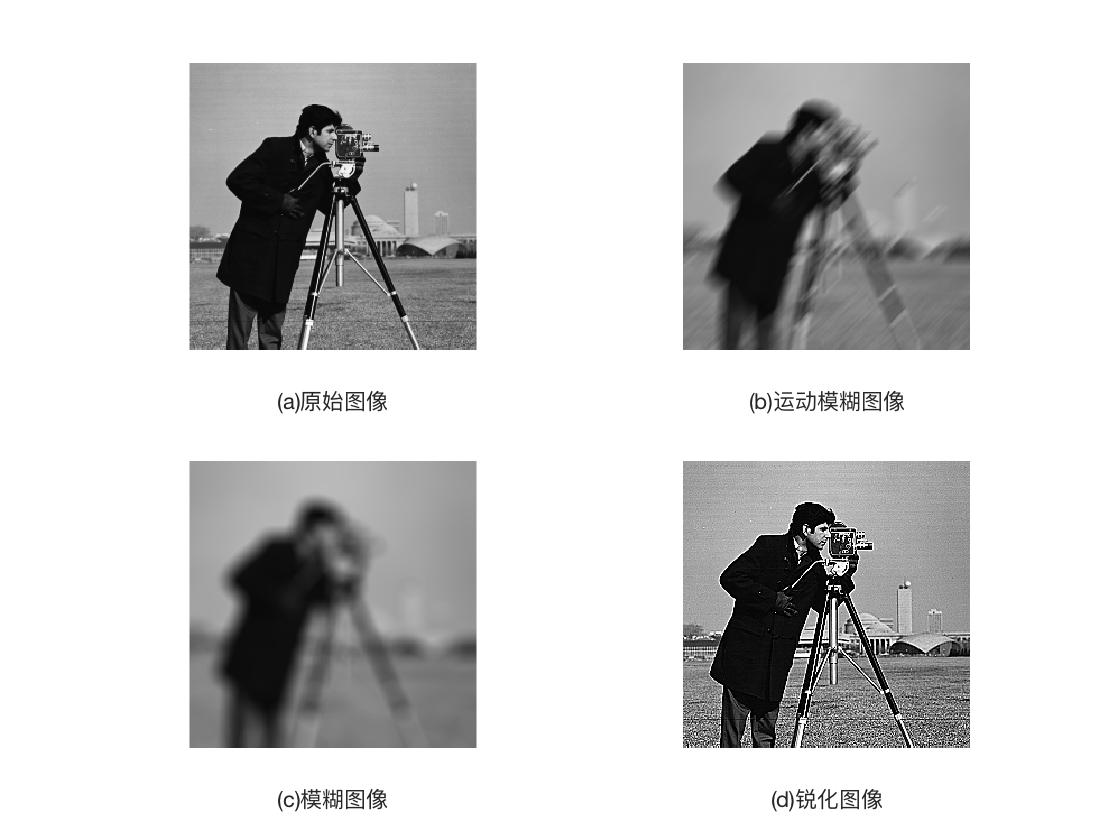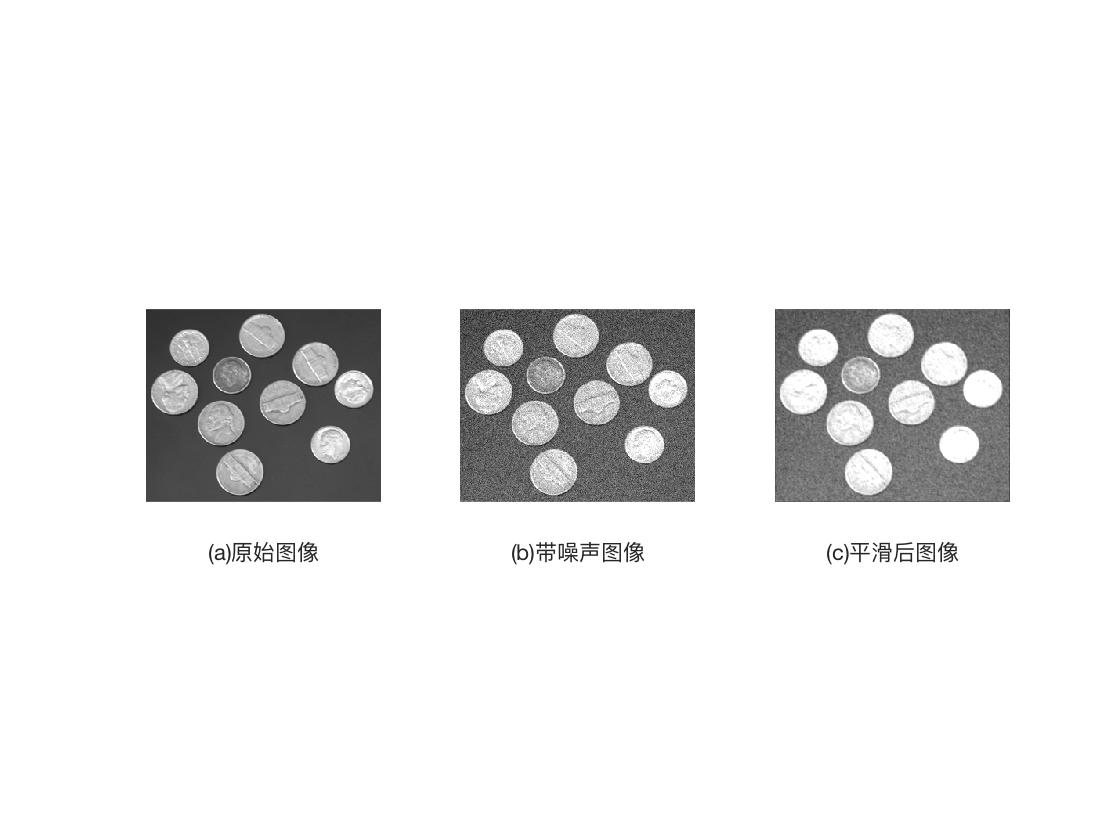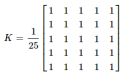2018-11-01 20:52:00 weixin_43418716 阅读数 10753
• ###### 容器技术助力企业应用平滑上云案例分享

越来越多的企业选择用Docker作为上云的迁移方案，如何让上云的过程更加平滑无缝，如何支持或适配不同的日志、监控、存储、部署能力成了一个棘手的问题。本次分享主要聚焦上述问题进行探讨。

3433 人正在学习 去看看 CSDN讲师

# 平滑滤波——matlab图像处理

MATLAB中提供的imfliter函数用于实现图像的平滑处理，其调用格式如下。
B=imfliter（A，H）：使用多维滤波器H对图像A进行滤波（平滑）。参数A可以是任意维的二值或非奇异数值型矩阵。参数H为矩阵，表示滤波器。H常由函数fspecial输出得到。返回值B与A的维数相同。
B=imfliter（A,H，optional1，optional2，…）

X 输入图像的外边界通过X来扩展，X默认值为0
symmetric 输入图像的外部边界通过镜像反射其内部边界来扩展
circular 输入图像的别界通过假设输入图像为周期函数来扩展
relicate 输入图像的外部别界通过复制内部别界的值来扩展
same 输入和输出图像大小相等，默认操作
full 输出图像比输入图像大
corr 使用相关进行滤波（平滑）
conv 使用卷积进行滤波（平滑）

matlab中提供的fspecial函数用于创建二维滤波器：
h=fspecial（type）：
type可以是：average，disk，gaussian，laplacian，log，motion，prewitt，sobel，unsharp。

h=fspecial（type，parameters）：创建指定类型和指定参数的二维滤波器h。参数parameters为与滤波器有关的参数。

clear all;
subplot(2,2,1);imshow(I);
xlabel('(a)原始图像');
H = fspecial('motion',20,45);
MotionBlur = imfilter(I,H,'replicate');
subplot(2,2,2);imshow(MotionBlur);
xlabel('(b)运动模糊图像');
H=fspecial('disk',10);
blurred = imfilter(I,H,'replicate');
subplot(2,2,3);imshow(blurred);
xlabel('(c)模糊图像');
H=fspecial('unsharp');
sharpened = imfilter(I,H,'replicate');
subplot(2,2,4);imshow(sharpened);
xlabel('(d)锐化图像');clear all;
Inoised = imnoise(I,'gaussian',0.1,0.005);%对图像进行高斯噪声加噪
%制定卷积核
h=ones(3,3)/5;
h(1,1) = 0;
h(1,3) = 0;
h(3,1) = 0;
h(1,3) = 0;
%平滑运算
I2=imfilter(Inoised,h);
subplot(1,3,1);imshow(I);
xlabel('(a)原始图像');
subplot(1,3,2);imshow(Inoised);
xlabel('(b)带噪声图像');
subplot(1,3,3);imshow(I2);
xlabel('(c)平滑后图像');2013-04-10 14:51:37 mlkiller 阅读数 4225
• ###### 容器技术助力企业应用平滑上云案例分享

越来越多的企业选择用Docker作为上云的迁移方案，如何让上云的过程更加平滑无缝，如何支持或适配不同的日志、监控、存储、部署能力成了一个棘手的问题。本次分享主要聚焦上述问题进行探讨。

3433 人正在学习 去看看 CSDN讲师

# 一含义

模糊（平滑）是一种常用的图片处理方式，它的作用可以用来降低噪声，还有其他用途

看一下opencv 里面的公式g(i,j)是目标坐标的像素值， f(i+k,j+l)是k,l这些地方的像素值， h(k,l)是 kernel,  我不知道怎么去准确翻译它的意义，它是过滤器的系数。

简单的按照我的思路去理解，就是一个权值，模糊的含义是将所有的像素按照一定的权值进行运算，得到一个比较均衡的结果。

# 三 算法

1 均值模糊
均值模糊很简单就是周边所有的影响都是1，求平均值即可2 高斯模糊# 四均值模糊的代码和效果

先放上均值模糊的代码
void boxblur(Mat input ,Mat &out, int x, int y)
{
// accept only char type matrices
CV_Assert(input.depth() != sizeof(uchar));

out.create(input.size(),input.type());

int nChannels = input.channels();
int nRows = input.rows;
int nCols = input.cols;

int size = x * y;
float kernel = 1.0/size;

int i,j;
uchar* p;
uchar* q;
uchar R,G,B;

for( i = x; i < nRows - x; ++i)
{
q = out.ptr<uchar>(i);
for ( j = y; j < nCols - y; ++j)
{
float sumR = 0;
float sumG = 0;
float sumB = 0;
for (int k =0; k<x;k++)
{
p = input.ptr<uchar>(i-x+k);
for(int l = 0; l < y;l++)
{
sumB += input.at<uchar>(i - x + k,(j + l - y)*nChannels) * kernel;//p[(l + j -y)*nChannels ] * kernel;
sumG += input.at<uchar>(i - x + k,(j + l - y)*nChannels + 1) * kernel;//p[(l + j -y)*nChannels + 1] * kernel;
sumR += input.at<uchar>(i - x + k,(j + l - y)*nChannels + 2) * kernel;//p[(l + j -y)*nChannels + 2] * kernel;
}
}
q[j*nChannels] = sumB;
q[j*nChannels+1] = sumG;
q[j*nChannels+2] = sumR;
}
}

}原始 opencv 本文# 五高斯模糊的代码和效果

void gaussblur(Mat input ,Mat &out, int x, int y)
{
float sigma = 1.5;
Mat kernel;
float pi = 3.1415926;

kernel.create(x ,y ,CV_32F);

float mx = x/2.0;
float my = y/2.0;


       //这里有问题，后面做修正。
for (int i =0; i< x;i++)
{
for (int j =0; j<y;j++)
{
kernel.at<float>(i,j) = exp(-1 * ((i - mx) * (i - mx) +(j - my) * (j-my) )/( 2 * sigma * sigma))/(2 * pi * sigma *sigma) ;
}
}


int nChannels = input.channels();
int nRows = input.rows;
int nCols = input.cols;

out.create(input.size(),input.type());
uchar* p;
uchar* q;
float* s;

for(int  i = x; i < nRows - x; ++i)
{
q = out.ptr<uchar>(i);
for (int j = y; j < nCols - y; ++j)
{
float sumR = 0;
float sumG = 0;
float sumB = 0;
for (int k =0; k<x;k++)
{
p = input.ptr<uchar>(i-x+k);
s = kernel.ptr<float>(k);
for(int l = 0; l < y;l++)
{
sumB += p[(l + j -y)*nChannels ] * s[l];//input.at<uchar>(i - x + k,(j + l - y)*nChannels) * kernel;//
sumG += p[(l + j -y)*nChannels + 1] *s[l];//input.at<uchar>(i - x + k,(j + l - y)*nChannels + 1) * kernel;//
sumR += p[(l + j -y)*nChannels + 2] * s[l];//input.at<uchar>(i - x + k,(j + l - y)*nChannels + 2) * kernel;
}
}
q[j*nChannels] = sumB;
q[j*nChannels+1] = sumG;
q[j*nChannels+2] = sumR;
}
}

}原始 opencv 本文float sum = 0;
for (int i =0; i< x;i++)
{
for (int j =0; j<y;j++)
{
sum+= kernel.at<float>(i,j) = exp(-1 * ((i - mx) * (i - mx) +(j - my) * (j-my) )/( 2 * sigma * sigma))/(2 * pi * sigma *sigma) ;
}
}
for (int i =0; i< x;i++)
{
for (int j =0; j<y;j++)
{
kernel.at<float>(i,j) = kernel.at<float>(i,j)/ sum ;
}
}

sigma = 0.3*((ksize-1)*0.5 - 1) + 0.8 .

2018-05-15 14:09:16 Blaze_bxh 阅读数 1802
• ###### 容器技术助力企业应用平滑上云案例分享

越来越多的企业选择用Docker作为上云的迁移方案，如何让上云的过程更加平滑无缝，如何支持或适配不同的日志、监控、存储、部署能力成了一个棘手的问题。本次分享主要聚焦上述问题进行探讨。

3433 人正在学习 去看看 CSDN讲师

1. 使用不同的低通滤波器模糊图像；

2. 对图像使用定制化滤波器；

## 2D卷积

Opencv提供的接口函数cv2.filter2D()来对图像做卷积。我们定义一个5x5的均值滤波器核，如下：## 图像模糊（图像平滑）

Opencv提供了四种图像模糊的技术。

### 1. Averaging

Opencv 接口：cv2.blur(), cv2.boxFilter(),只需要指定卷积核的宽高即可。

### 4. Bilateral Filtering

2013-06-28 10:58:42 diarymaggie 阅读数 208
• ###### 容器技术助力企业应用平滑上云案例分享

越来越多的企业选择用Docker作为上云的迁移方案，如何让上云的过程更加平滑无缝，如何支持或适配不同的日志、监控、存储、部署能力成了一个棘手的问题。本次分享主要聚焦上述问题进行探讨。

3433 人正在学习 去看看 CSDN讲师

#include "cv.h"
#include "highgui.h"

using namespace cv;

#define PI 3.1415926

int smooth_box={1,1,1,1,1,1,1,1,1};
int smooth_gauss={1,2,1,2,4,2,1,2,1};
int sharpen_laplacian={-1,-1,-1,-1,9,-1,-1,-1,-1};

//平滑和锐化
Mat TemplateOP(Mat& mat)
{
int M=mat.rows;
int N=mat.cols;

float coef;
int coefArray;

#if 0
coef=(float)(1.0/9.0);
memcpy(coefArray,smooth_box,sizeof(smooth_box));
#elif 0
coef=(float)(1.0/16.0);
memcpy(coefArray,smooth_gauss ,sizeof(smooth_gauss));
#else
coef=(float)1.0;
memcpy(coefArray,sharpen_laplacian,sizeof(sharpen_laplacian));
#endif

Mat ret(M,N,CV_8U);
for(int i=1;i<M-1;i++)
{
for(int j=1;j<N-1;j++)
{
float tempNum=0.0;
int k=0;
for(int i1=-1;i1<=1;i1++)
for(int j1=-1;j1<=1;j1++)
tempNum+=mat.ptr<uchar>(i+i1)[j+j1]*coefArray[k++];
tempNum*=coef;
uchar temp;
if(tempNum>255.0) temp=255;
else if(tempNum<0.0) temp=0;
else temp=(uchar)tempNum;

ret.ptr<uchar>(i)[j]=temp;
}
}

return ret;
}

//中值滤波
Mat MedianFilter(Mat& mat)
{
int M=mat.rows;
int N=mat.cols;

vector<int> nums(9,0);
Mat ret(M,N,CV_8U);
for(int i=1;i<M-1;i++)
{
for(int j=1;j<N-1;j++)
{
int k=0;
for(int i1=-1;i1<=1;i1++)
for(int j1=-1;j1<=1;j1++)
nums[k++]=mat.ptr<uchar>(i+i1)[j+j1];
sort(nums.begin(),nums.end());
ret.ptr<uchar>(i)[i]=nums;
}
}

return ret;
}

int main()
{
//读取图像
Mat image;

int M=image.rows;
int N=image.cols;

//几何变换

Mat newImage=TemplateOP(image);

cvNamedWindow("test",CV_WINDOW_AUTOSIZE);
imshow("test",newImage);

waitKey(0);
return 0;
}

2018-03-18 14:51:58 qq_27591163 阅读数 2063
• ###### 容器技术助力企业应用平滑上云案例分享

越来越多的企业选择用Docker作为上云的迁移方案，如何让上云的过程更加平滑无缝，如何支持或适配不同的日志、监控、存储、部署能力成了一个棘手的问题。本次分享主要聚焦上述问题进行探讨。

3433 人正在学习 去看看 CSDN讲师

1 1 1                    1   2   1

1/8  （ 1 1 1）     1/16 （2  4   2）

1 1 1                   1    2   1

{
int avg = 0;
BYTE * m_pdata;
int width, height;
m_pdata = getData();
width = getWidth();
height = getHeight();
BYTE* temp = new BYTE[width *height];
memset(temp, 255, width*height);
for (int j = 1; j<height - 1; j++)
for (int i = 1; i < width - 1; i++)
{
avg = (int)((m_pdata[(j - 1)*width + (i - 1)] + m_pdata[(j - 1)*width + i] + m_pdata[(j - 1)*width + (i + 1)]
+ m_pdata[j*width + (i - 1)] + m_pdata[j*width + (i + 1)] + m_pdata[(j + 1)*width + (i - 1)] + m_pdata[(j + 1)*width + i]
+ m_pdata[(j + 1)*width + (i + 1)]) / 8);
temp[width*j + i] = avg;

}
memcpy(m_pdata, temp, width*height);
delete temp;
} 

	if (n >= 3 && n % 2 == 1)
{
BYTE * temp = new BYTE[width*height];
memset(temp, 255, width*height);
n2 = (n - 1) / 2;
int chou = (n+n-1) / 2;
for(int j=n2;j<=height-n2;j++)
for (int i = n2; i < width - n2; i++)
{
int m = 0;
for (int yy = j - n2; yy <= j + n2; yy++)
{
modo[m++] = p_data[yy*width + i];
}
for (int xx = i - n2; xx <= i + n2; xx++)
{
modo[m++] = p_data[j*width + xx];
}
std::sort(modo, modo + m);
medi = modo[chou];
temp[j*width + i] = medi;
}
memcpy(p_data, temp, width*height);
delete temp;
}